## Transistor Hybrid Model:

The basic assumption in arriving at a transistor linear model or equivalent circuit is that the variations about the operating or quiescent point are small and, therefore, the transistor parameters can be considered constant over the small range of operation.

Many transistor models have been proposed, each one hav­ing its particular merits and demerits. The transistor model presented here, is given in terms of the h parameters, which are real numbers at audio frequencies, are easy to measure, can also be obtained from the static characteristics of a transistor, and are particularly convenient to use in analysis and design of circuit. Furthermore, a set of h parameters is specified for many transis­tors by the manufacturers.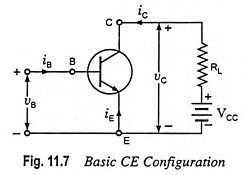To derive a hybrid model for a transistor, let us consider the basic CE amplifier circuit given in Fig. 11.7. The variables iB, iC, vB and vC represent the total instantaneous values of currents and voltages. We may select the input current iB and output voltage vC as independent variables. Since input voltage vB is some function f1 of iB and vC and output current iC is another function f2 of iB and vC, we may write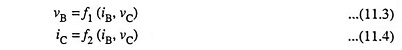Making a Taylor’s series expansion of Eqs. (11.3) and (11.4) about the zero signal operating point (IB, VC) and neglecting higher-order terms we have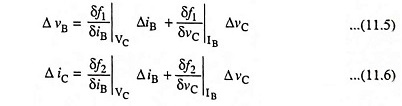where partial derivatives δf1/δiB and δf2/δiB are taken, keeping collector voltage VC constant while partial derivates δf1/δvC and δf2/δvC are taken, keeping base current IB constant.

The quantities ΔvB, ΔvC, ΔiB and ΔiC represent the small-signal (incremental) base and collector voltages and currents and may be represented as vb, vc, ib, and ic respectively as per standard notations. We may now write Eqs. (11.5) and (11.6) as below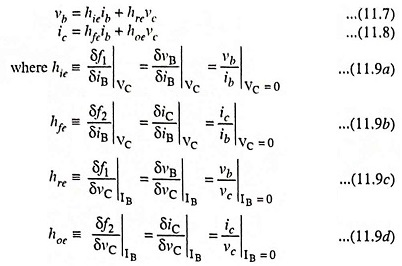The partial derivatives of Eq. (11.9) define the h parameters for the transistor in common-emitter (CE) configuration.

Equations (11.7) and (11.8) are found to be of exactly the same form as Eqs. (11.1) and (11.2) hence, the model shown in Fig. 11.4 can be used to represent a transistor.

The common-emitter (CE), common-base (CB), and common-collector (CC) configurations, their Transistor Hybrid Model and their terminal volt-ampere equations are summarized in Table 11.2.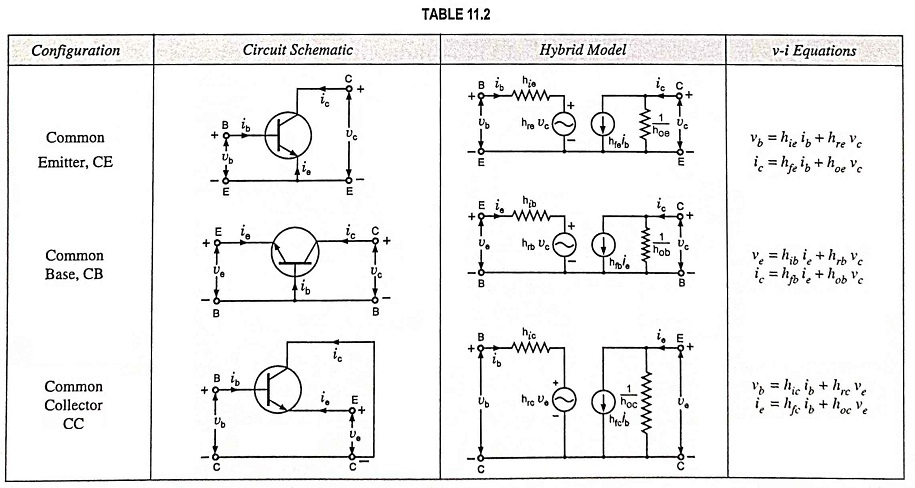The circuits and equations in Table 11.2 are valid for either an N-P-N or P-N-P transistor and are independent of the type of load or biasing method.

Scroll to Top